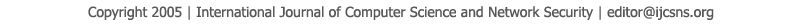To search, Click below search items.All Published Papers Search Service
 Title Author Year Keyword
 Title Statistical Prediction Based on Estimation of Conditional Density Author K Koteswara Rao, P.Veerabhadra Rao, V.Venkat, GSVP Raju Citation Vol. 8  No. 4  pp. 198-211 Abstract Many real problems such as stock market prediction, weather forecasting etc has inherent randomness associated with them. Adopting a probabilistic framework for prediction can accommodate this uncertain relationship between past and future. Typically the interest is in the conditional probability density of the random variable involved. One approach for prediction is with time series and auto regression models. In this work, liner prediction method and approach for calculation of prediction coefficient are given and probability of error for different estimators is calculated. The existing techniques all require in some respect estimating a parameter of some assumed solution. So, an alternative approach is proposed. The alternative approach is to estimate the conditional density of the random variable involved. The approach proposed in this thesis involves estimating the (descretized) conditional density using a Markovian formulation when two random variables are statistically dependent, knowing the value of one of them lets us get a better estimate of the value of the other one. The conditional density is estimated as the ratio of the two dimensional joint density to the one-dimensional density of random variable whenever the later is positive. Markov models are used in the problems of making a sequence of decisions and problem that have an inherent temporality that is consisting of a process that unfolds in time in time. In the continuous time Markov chain models the time intervals between two consecutive transitions may also be a continuous random variable. The Markovian approach is particularly simple and fast for almost all classes of classes of problems requiring the estimation of conditional densities. Keywords Statistical prediction, unbiased ness, Sufficiency, smoothing, univariate time series, Autoregressive, Markov chains URL http://paper.ijcsns.org/07_book/200804/20080429.pdf